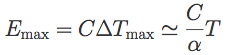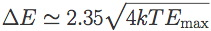# Transition Edge Sensors (TES)A transition-edge sensor is a thermometer made from a superconducting film operated near its transition temperature Tc. While in its transition from superconductor to normal metal, a very small change in temperature causes a large change in resistance. Transition widths are typically in the order of a milli-Kelvin. Many different type of superconducting metal films are used by different groups as TESs. We use thin Molybdenum/Gold bilayers for our devices. Our Mo/Au TESs use the proximity effect, where the two metals behave as a single film with a transition temperature between 800 mK (Mo's Tc) and 0 K (Au's Tc). Typically our films are tuned for a Tc = 100 mK. The low temperature is needed because the energy resolution of these devices scales with temperature, so cryogenic temperatures are need for high-energy resolution. The figure of merit for microcalorimeter resistive thermometers is a parameter called α, defined aswhere T is the temperature and R is the resistance of the TES. The energy resolution of a TES can be approximated bywhere k is Boltzmann's constant, T is the TES temperature, C is the microcalorimeter's heat capacity, α was define above, and the factor of 2.35 is to convert standard deviation into full-width at half maximum (FWHM) (just an easier way to measure the resolution of our devices). So for good energy resolution, we want to operate the devices as cold as we can, have a low heat capacity and a high α.

An important limitation is the fact that the TES operates well as a thermometer only in its transition. Once it goes normal, its resistance no longer changes with temperature (much), and
α 0. This implies a maximum temperature change in the TES, which in turn implies a maximum energy of the incoming photons:So the parameters C and α are tied by the desired energy bandpass of the microcalorimeter. We can then state the theoretical energy resolution of a microcalorimeter in a simple formula that only depends on the temperature of operation and the bandpass of the device:For a typical X-ray application, the bandpass is from ~ 0.1 keV to 10 keV, and operating at 100 mK gives us an energy resolution of ΔE = 1.4 eV FWHM, close to two orders of magnitude better than the theoretical limits for X-ray CCDs.This is a picture of an 8 x 8 array of TES microcalorimeters built at the NASA Goddard Space Flight Center. Each Pixel is 250 μm on a side and has a Mo/Au TES and a Bi/Cu absorber to obtain 95% X-ray stopping efficiency at 10 keV. Our challenge and goal is to build much larger arrays (tens of thousands of pixels) while striving for the best energy resolution possible (4 eV or better).